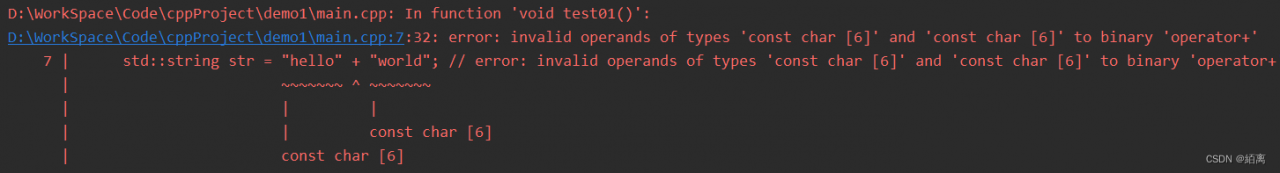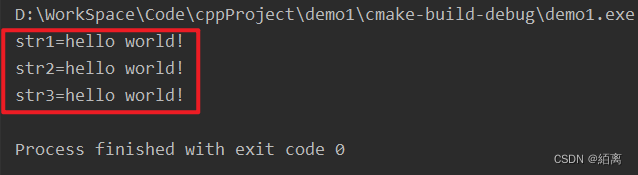# [Solved] error: invalid operands of types ‘const char ‘ and ‘const char ‘ to binary ‘operator+‘

preface

When using strings in C++, we habitually use `+` to connect two strings enclosed in `""`. The error is reported: `error: invalid operators of types' const char  'and' const char  'to binary' operator+'`,

1. Scenarios``````	//std::string str = "hello" + "world"; // error: invalid operands of types 'const char ' and 'const char ' to binary 'operator+'
//std::string str = std::string("hello") + " world"; // correct
//std::string str = "hello" + std::string("world"); //correct
std::string str = "hello"" world"; //correct

cout << "str=" << str << endl;
``````

When using the+operator to connect two strings wrapped with `""`, an error occurs. The reason is that in C++, the strings enclosed by `""` are regarded as `const char* types`, rather than string types.

2. Solution

Refer to the explanation on stackoverflow. For details, please read error: invalid operators of types’ const char * ‘and’ const char * ‘to binary’ operator+’

1. Explicitly declare one of them as std::string type (recommended)

``````std::string str = std::string("hello") + " world";
``````

2. Remove the+and let the compiler splice strings (not recommended)

``````std::string str = "hello"" world";
``````

Most compilers automatically splice strings enclosed by `""`, but it is not guaranteed that all compilers are normal. It is recommended to display strings declared as string types and then perform the+operation.

3. Validation

``````	std::string str1 = std::string("hello") + " world" + "!"; // correct
std::string str2 = "hello" + std::string(" world") + "!"; //correct
std::string str3 = "hello"" world" "!"; //correct

cout << "str1=" << str1 << endl;
cout << "str2=" << str2 << endl;
cout << "str3=" << str3 << endl;
``````